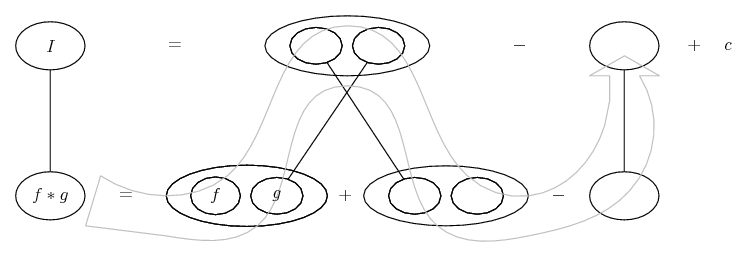# Thread: help with integral

1. ## help with integral

Hey,

I was going through my textbook, and came across this challenge question:

Solve $\displaystyle \int{\cos^2{x}dx}$ without using the half-angle formula.

I've been running through every trig identity I know, trying to figure out a way to simplify this. Does anyone have an idea? It's trivial to solve using the half-angle formula. I've been racking my brain and can't figure out a way to solve the question without it.

2. What about $\displaystyle \cos^2{x} = \frac{1}{2} + \frac{1}{2}\cos{2x}$? The double angle formula...

3.Originally Posted by centenialHey,

I was going through my textbook, and came across this challenge question:

Solve $\displaystyle \int{\cos^2{x}dx}$ without using the half-angle formula.

I've been running through every trig identity I know, trying to figure out a way to simplify this. Does anyone have an idea? It's trivial to solve using the half-angle formula. I've been racking my brain and can't figure out a way to solve the question without it.
Integration by parts.

4. Either method will work. I suspect you will find using $\displaystyle cos^2(x)= (1/2)(1+ cos(2x))$ easier.

5. Thanks! I guess I was confusing the double and half/angle formulas in my head. That makes sense, pretty straightforward to solve using the double angle.

mr. fantastic, is there a way to solve that using only integration by parts (without the double angle formula)? If so, I'd be very curious to see how to do that. Cos/sin don't get any simpler when you integrate/differentiate them, so I'm curious to see how that would work.

Thanks again guys.6.Originally Posted by centenialThanks! I guess I was confusing the double and half/angle formulas in my head. That makes sense, pretty straightforward to solve using the double angle.

mr. fantastic, is there a way to solve that using only integration by parts (without the double angle formula)? If so, I'd be very curious to see how to do that. Cos/sin don't get any simpler when you integrate/differentiate them, so I'm curious to see how that would work.

Thanks again guys.First we'll define our question as being I: $\displaystyle I = \int \cos^2(x)\,dx$ (this is important later)

$\displaystyle u = \cos(x) \: \rightarrow \: u' = -\sin(x)$

$\displaystyle v' = \cos(x) \: \rightarrow \: v = \sin(x)$

Integrating by parts: $\displaystyle \int u\,dv = uv - \int v\,du$

$\displaystyle I = \sin(x) \cos(x) + \int \sin(x) \sin(x)$

That second integral means you have to integrate by parts again

$\displaystyle p = \sin(x) \: \rightarrow \: p' = \cos(x)$

$\displaystyle q' = \sin(x) \: \rightarrow \: q = -\cos(x)$

Thus:

$\displaystyle -\sin(x) \cos(x) + \int \cos^2(x)$

Of course, we earlier defined $\displaystyle I = \int cos^2(x)$ - so substitute it in to remove the integral sign

$\displaystyle I = \sin(x) \cos(x) - (-\sin(x) \cos(x) + I)$

I'll leave you to finish that up, all it needs is algebraic manipulation

7.Originally Posted by e^(i*pi)That second integral means you have to integrate by parts again
Slightly easier actually to apply pythag at this point. Just in case a picture helps...... where (key in spoiler) ...

Spoiler:... is the product rule. Straight continuous lines differentiate downwards (integrate up) with respect to x. And,... is lazy integration by parts, doing without u and v.

Similar here: http://www.mathhelpforum.com/math-he...ts-154017.html
_________________________________________

Don't integrate - balloontegrate!

Balloon Calculus; standard integrals, derivatives and methods

Balloon Calculus Drawing with LaTeX and Asymptote!

#### Search Tags

integral